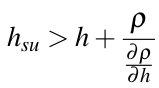# Enthalpy Limiter

The enthalpy limiter method is a method originally proposed by Schulze et al. and implemented in the ThermoCycle library.

This method focuses on one-phase and two-phase fluid flow with 1-D spatial discretization according to the Finite Volume Method. In this type of model, there are points where the resulting set of equations is not solvable and simulation fails. This can typically occur in case of flow reversal, when the entering enthalpy is below a certain limit.

As shown by Schulze et al., a solvability criterion can be expressed in the following manner:where hsu is the entering enthalpy and h the enthalpy in a cell. The partial derivative is a negative term whereas the density is positive.

This inequality states that in cases of flow reversal, an unsolvable system of equations appears, if the enthalpy of the entering fluid is below a certain limit.

To avoid this, the enthalpy limiter method is implemented: in this approach, the enthalpy signal transmitted by the stream connectors is limited by a lower boundary, ensuring the system remains solvable in all cases.

To activate the method in ThermoCycle, the Cell1Dim and Flow1Dim components must be replaced by the Cell1dim_limit and Flow1Dim_limit equivalent components.

# Reference

Christian Schulze, Manuel Graber, and Wilhelm Tegethoff. A limiter for preventing singularity in simplified finite volume methods. In Mathematical Modelling, 2012.